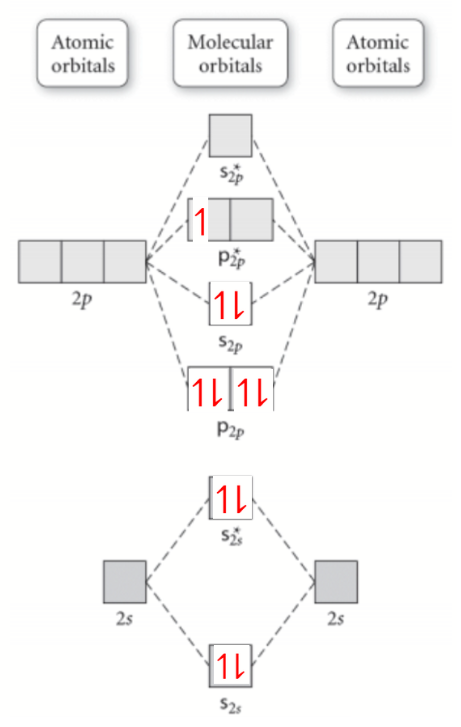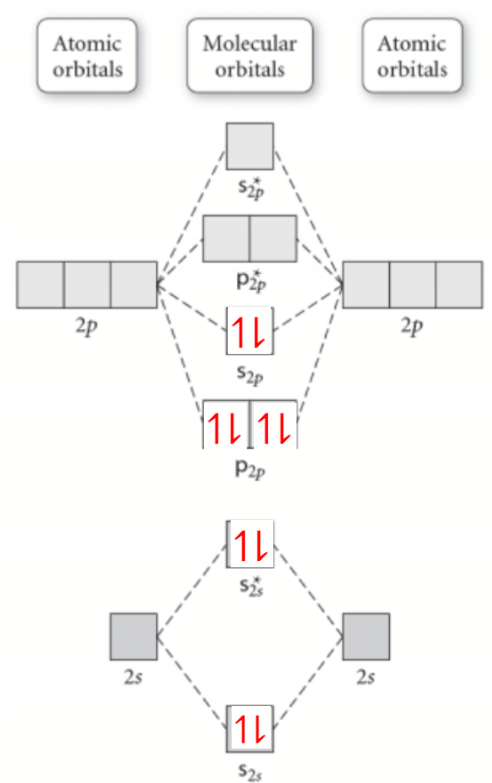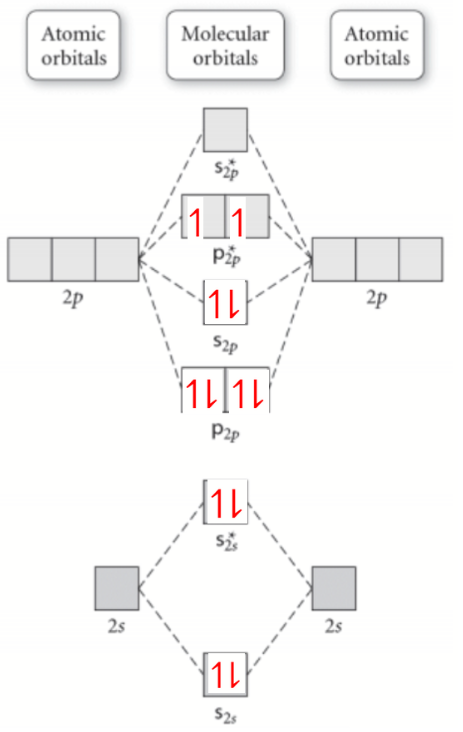# Problem: Predict the order of the N--O bond strengths in NO, NO+, and NO-, and describe the magnetic properties of each.

###### FREE Expert Solution

We are asked to predict the order of the N--O bond strengths in NO, NO+, and NO-, and describe the magnetic properties of each.

First identify the number of valence electrons for each. The number of valence electron per element is based on the group number. A (+) charge means we need to subtract and electron and (-) means we need to add.

A) NO

(VE N) + (VE O) = (5 e-) + (6 e-) = 11 e

B) NO+

11 e- 1 e- = 10 e-

C) NO

11 e+ 1 e- = 12 e-

Next, fill the molecular orbitals (middle part) with the valence electrons for each.

A) NOB) NO+C) NO-Next count electrons on the bonding (no star) and anti-bonding (with star) orbitals.

A) NO

Bonding = 8 e-

Anti-Bonding = 3 e

B) NO+

Bonding = 8 e-

Anti-Bonding = 2 e

C) NO+

Bonding = 8 e-

Anti-Bonding = 4 e

Finally, use the equation for bond order:

A) NO

Bonding = 8 e-

Anti-Bonding = 3 e

Bond Order = 2.5 e-

B) NO+

Bonding = 8 e-

Anti-Bonding = 2 e

Bond Order = 3 e-

99% (73 ratings)###### Problem Details

Predict the order of the N--O bond strengths in NO, NO+, and NO-, and describe the magnetic properties of each.